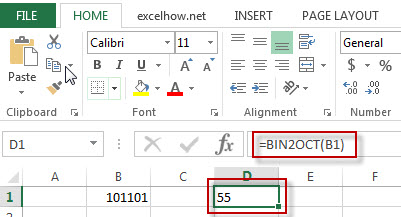# Excel Bin2Oct Function

This post will guide you how to use Excel BIN2OCT function with syntax and examples in Microsoft excel.

### Description

The Excel BIN2OCT function converts a binary number to octal.

The BIN2OCT function is a build-in function in Microsoft Excel and it is categorized as an Engineering Function.

The BIN2OCT function is available in Excel 2016, Excel 2013, Excel 2010, Excel 2007, Excel 2003, Excel XP, Excel 2000, Excel 2011 for Mac.

### Syntax

The syntax of the BIN2OCT function is as below:

= BIN2OCT (binary_number)

Where the BIN2OCT function argument is:
Binary_number -This is a required argument. The binary number that you want to convert to octal.

### Example

The below examples will show you how to use Excel BIN2OCT Function to convert a binary number to a octal number.

#1 = BIN2OCT (B1)